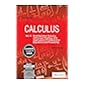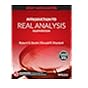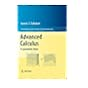Normal view MARC view ISBD view

# Introduction to real analysis / Robert G. Bartle, Donald R. Sherbert.

Material type:TextPublisher: New Delhi : Wiley India, c2011Edition: 4th ed.Description: xiii, 402 p. : Rs. 479.00 ill. ; 26 cm.ISBN: 9788126551811 (pbk); 0471433314 (hardback).Subject(s): Mathematical analysis | Functions of real variablesDDC classification: 515
Contents:
Ch. 1.Preliminaries: 1.1. Sets and functions; 1.2. Mathematical induction; 1.3. Finite and infinite sets -- Ch. 2. The Real Numbers: 2.1. The algebraic and order properties of R; 2.2. Absolute value and real line; 2.3. The completeness property of R; 2.4. Applications of the supremum property; 2.5. Intervals -- Ch. 3. Sequences and series: 3.1. Sequences and their limits; 3.2. Limit theorems; 3.3. Monotone sequences; 3.4. Subsequences and the Bolzano-Weierstrass theorem; 3.5. The Cauchy criterion; 3.6. Properly divergent sequences; 3.7. Introduction to infinite series -- Ch. 4. Limits: 4.1. Limits of functions; 4.2. Limit theorems; 4.3. Some extensions of the limit concept -- Ch. 5. Continuous functions: 5.1. Continuous runctions; 5.2 . Combinations of continuous runctions; 5.3. Continuous functions on intervals; 5.4. Uniform continuity; 5.5. Continuity and gauges; 5.6. Monotone and inverse functions -- Ch. 6. Differentiation: 6.1. The derivative; 6.2. The mean value theorem; 6.3. L'Hospital's rules; 6.4. Taylor's Theorem -- Ch. 7. The Riemann integral: 7.1. Riemann integral; 7.2. Riemann integrable functions; 7.3. The fundamental theorem; 7.4. The Darboux integral; 7.5. Approximate integration -- Ch. 8. Sequences of functions: 8.1. Pointwise and uniform convergence; 8.2. Interchange of limits; 8.3. The exponential and logarithmic functions; 8.4. The trigonometric functions -- Ch. 9. Infinite series: 9.1. Absolute convergence; 9.2. Tests for absolute convergence; 9.3. Tests for nonabsolute convergence; 9.4. Series of functions -- Ch. 10. The generalized Riemann integral: 10.1. Definition and main poperties; 10.2. Improper and Lebesgue integrals; 10.3. Infinite intervals; 10.4. Convergence theorems -- Ch. 11. A glimpse into topology: 11.1. Open and closed sets in R; 11.2 Compact sets; 11.3. Continuous functions; 11.4. Metrtic Spaces -- Appendix A. Logic and proofs -- Appendix B. Finite and countable sets -- Appendix C. The Riemann and Lebesgue criteria -- Appendix D. Approximate integration -- Appendix E. Two examples.
Summary: "This text provides the fundamental concepts and techniques of real analysis for students in all of these areas. It helps one develop the ability to think deductively, analyse mathematical situations and extend ideas to a new context. Like the first three editions, this edition maintains the same spirit and user-friendly approach with addition examples and expansion on Logical Operations and Set Theory. There is also content revision in the following areas: introducing point-set topology before discussing continuity, including a more thorough discussion of limsup and limimf, covering series directly following sequences, adding coverage of Lebesgue Integral and the construction of the reals, and drawing student attention to possible applications wherever possible"-- Provided by publisher.
List(s) this item appears in: 2015-09-25
Item type Current location Call number Status Date due Barcode Item holds
Book Chennai Mathematical Institute
General Stacks
515 BAR (Browse shelf) Available 9632
Book Chennai Mathematical Institute
General Stacks
515 BAR (Browse shelf) Available 9633
Total holds: 0
##### Browsing Chennai Mathematical Institute Shelves , Shelving location: General Stacks Close shelf browser515 APO Calculus/ Vol. II multi-variable calculus and linear algebra, with applications to differential equations and probability. 515 APO Calculus/ Vol. II multi-variable calculus and linear algebra, with applications to differential equations and probability. 515 APO Calculus/ Vol. II multi-variable calculus and linear algebra, with applications to differential equations and probability. 515 BAR Introduction to real analysis / 515 BAR Introduction to real analysis / 515 CAL Advanced calculus : a geometric view / 515 CON A course in abstract analysis /

Includes bibliographical references and index.

Ch. 1.Preliminaries: 1.1. Sets and functions; 1.2. Mathematical induction; 1.3. Finite and infinite sets -- Ch. 2. The Real Numbers: 2.1. The algebraic and order properties of R; 2.2. Absolute value and real line; 2.3. The completeness property of R; 2.4. Applications of the supremum property; 2.5. Intervals -- Ch. 3. Sequences and series: 3.1. Sequences and their limits; 3.2. Limit theorems; 3.3. Monotone sequences; 3.4. Subsequences and the Bolzano-Weierstrass theorem; 3.5. The Cauchy criterion; 3.6. Properly divergent sequences; 3.7. Introduction to infinite series -- Ch. 4. Limits: 4.1. Limits of functions; 4.2. Limit theorems; 4.3. Some extensions of the limit concept -- Ch. 5. Continuous functions: 5.1. Continuous runctions; 5.2 . Combinations of continuous runctions; 5.3. Continuous functions on intervals; 5.4. Uniform continuity; 5.5. Continuity and gauges; 5.6. Monotone and inverse functions -- Ch. 6. Differentiation: 6.1. The derivative; 6.2. The mean value theorem; 6.3. L'Hospital's rules; 6.4. Taylor's Theorem -- Ch. 7. The Riemann integral: 7.1. Riemann integral; 7.2. Riemann integrable functions; 7.3. The fundamental theorem; 7.4. The Darboux integral; 7.5. Approximate integration -- Ch. 8. Sequences of functions: 8.1. Pointwise and uniform convergence; 8.2. Interchange of limits; 8.3. The exponential and logarithmic functions; 8.4. The trigonometric functions -- Ch. 9. Infinite series: 9.1. Absolute convergence; 9.2. Tests for absolute convergence; 9.3. Tests for nonabsolute convergence; 9.4. Series of functions -- Ch. 10. The generalized Riemann integral: 10.1. Definition and main poperties; 10.2. Improper and Lebesgue integrals; 10.3. Infinite intervals; 10.4. Convergence theorems -- Ch. 11. A glimpse into topology: 11.1. Open and closed sets in R; 11.2 Compact sets; 11.3. Continuous functions; 11.4. Metrtic Spaces -- Appendix A. Logic and proofs -- Appendix B. Finite and countable sets -- Appendix C. The Riemann and Lebesgue criteria -- Appendix D. Approximate integration -- Appendix E. Two examples.

"This text provides the fundamental concepts and techniques of real analysis for students in all of these areas. It helps one develop the ability to think deductively, analyse mathematical situations and extend ideas to a new context. Like the first three editions, this edition maintains the same spirit and user-friendly approach with addition examples and expansion on Logical Operations and Set Theory. There is also content revision in the following areas: introducing point-set topology before discussing continuity, including a more thorough discussion of limsup and limimf, covering series directly following sequences, adding coverage of Lebesgue Integral and the construction of the reals, and drawing student attention to possible applications wherever possible"-- Provided by publisher.